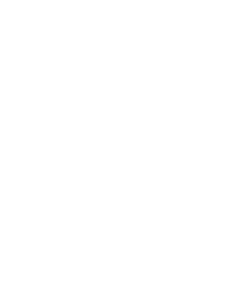October 12, 2023
October 12, 2023
October 12, 2023
###### Operating-Systems
October 12, 2023
 Question 17
What is the output of this C++ program?A 910 B 920 C 870 D 900
Question 17 Explanation:
Step-1: In main function, number variable has been initialized to 30.
Step-2: We pass address of number as parameters in the square function. As per the concept of function overloading in C++ the second square function will be executed.
Step-3: –(*y) will be executed first since decrement operator has the higher precedence than multiplication.
*x = (*x) * –(*y);
*x = 30 * 29;
*x = 870
Note: x is a pointer variable and it holds the address of the variable number.
Question 17 Explanation:
Step-1: In main function, number variable has been initialized to 30.
Step-2: We pass address of number as parameters in the square function. As per the concept of function overloading in C++ the second square function will be executed.
Step-3: –(*y) will be executed first since decrement operator has the higher precedence than multiplication.
*x = (*x) * –(*y);
*x = 30 * 29;
*x = 870
Note: x is a pointer variable and it holds the address of the variable number.